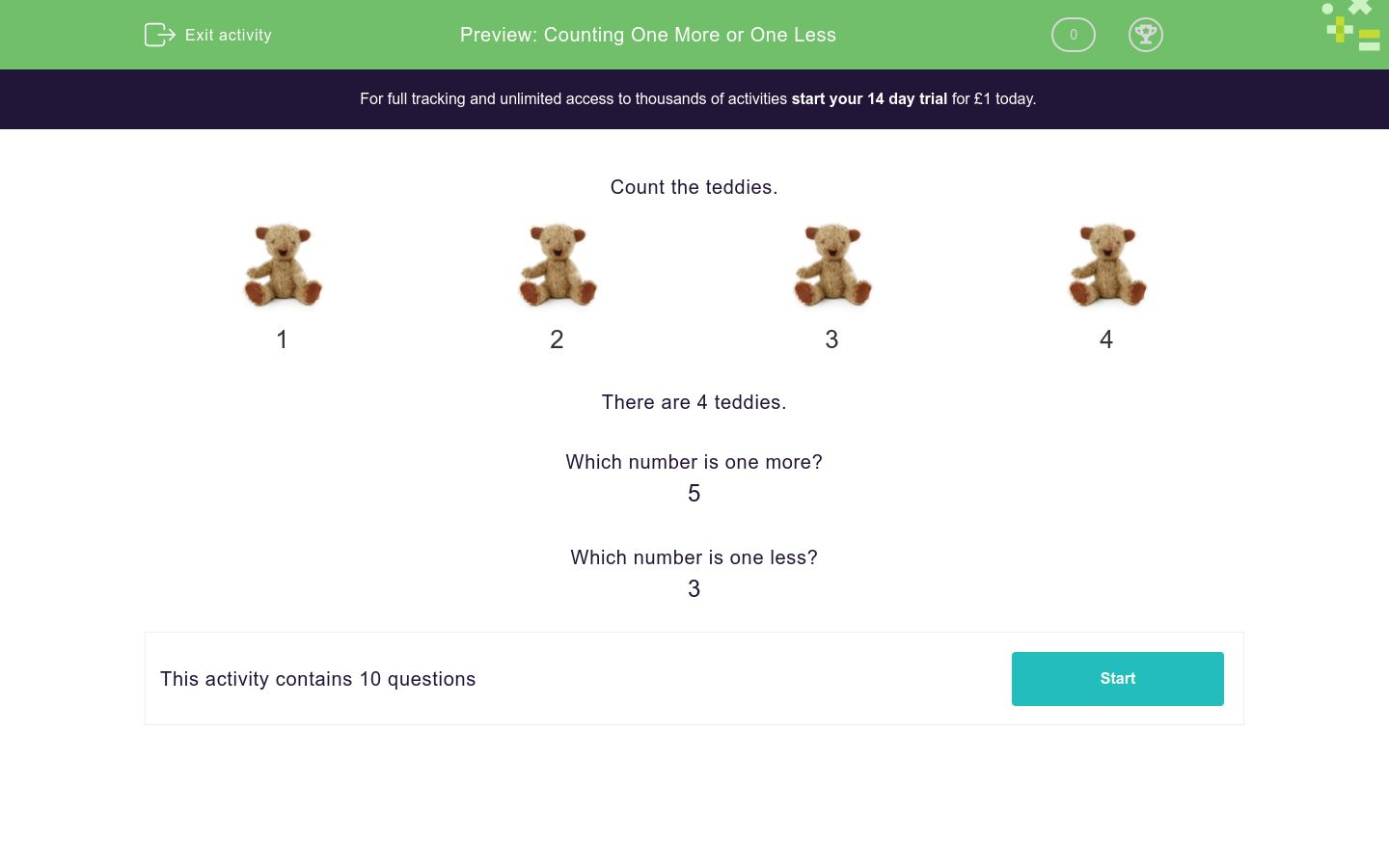# Counting One More or One Less

In this worksheet, children must state the number that is one more or one less.Key stage:  KS 1

Curriculum topic:   Number: Number and Place Value

Curriculum subtopic:   Identify One More/One Less

Difficulty level:### QUESTION 1 of 10

Count the teddies.1 2 3 4

There are 4 teddies.

Which number is one more?

5

Which number is one less?

3

Count the carrots.Choose the number which is one more.

8

6

7

Count the faces.Choose the number which is one less.

2

3

4

Find the number  5  on the number line.Choose the number which is one less.

6

4

5

Count the teddies.Choose the number which is one more.

5

4

6

Find the number  7  on the number line.Choose the number which is one more.

8

6

7

Write the number which is one more than

8

Count the balls.Choose the number which is one more.

8

9

10

Write the number which is one less than

8

Find the number  10  on the number line.Write the number which is one less.

Count the carrots.Choose the number which is one more.

8

6

7

• Question 1

Count the carrots.Choose the number which is one more.

7
EDDIE SAYS
1... 2... 3... 4... 5... 6 and then... 7
• Question 2

Count the faces.Choose the number which is one less.

2
EDDIE SAYS
1... 2... 3
• Question 3

Find the number  5  on the number line.Choose the number which is one less.

4
EDDIE SAYS
0... 1... 2... 3... 4... 5
• Question 4

Count the teddies.Choose the number which is one more.

6
EDDIE SAYS
1... 2... 3... 4... 5 and then... 6
• Question 5

Find the number  7  on the number line.Choose the number which is one more.

8
EDDIE SAYS
0... 1... 2... 3... 4... 5... 6... 7 and then... 8
• Question 6

Write the number which is one more than

8

9
EDDIE SAYS
0... 1... 2... 3... 4... 5... 6... 7... 8 and then... 9
• Question 7

Count the balls.Choose the number which is one more.

10
EDDIE SAYS
1... 2... 3... 4... 5... 6... 7... 8... 9 and then... 10
• Question 8

Write the number which is one less than

8

7
EDDIE SAYS
0... 1... 2... 3... 4... 5... 6... 7... 8
• Question 9

Find the number  10  on the number line.Write the number which is one less.

9
EDDIE SAYS
0... 1... 2... 3... 4... 5... 6... 7... 8... 9... 10
• Question 10

Count the carrots.Choose the number which is one more.

6
EDDIE SAYS
1... 2... 3... 4... 5 and then... 6
---- OR ----

Sign up for a £1 trial so you can track and measure your child's progress on this activity.

### What is EdPlace?

We're your National Curriculum aligned online education content provider helping each child succeed in English, maths and science from year 1 to GCSE. With an EdPlace account you’ll be able to track and measure progress, helping each child achieve their best. We build confidence and attainment by personalising each child’s learning at a level that suits them.

Get started